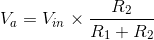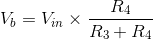Wheatstone Bridge

Working principle

The Wheatstone Bridge is a simple circuit for measuring an unknown resistance with the help of three other resistors and an input voltage. It finds an application in many measurement circuits and instruments.

Since the resistor pairs R1 and R2, R3 and R4 are in parallel with each other and the voltage source Vin, we can use the voltage divider equation to identify the voltage difference V- V= Vab.Substituting values gives us that R= 996 Ohms.

Useful formulaExperiment

Balance the bridge by making R= 2 Ohms, R= 1 Ohm and R= 1992 Ohms. What is Vab now? Why?